Courses

# Facts That Matter- Statistics Class 10 Notes | EduRev

## Class 10 : Facts That Matter- Statistics Class 10 Notes | EduRev

The document Facts That Matter- Statistics Class 10 Notes | EduRev is a part of the Class 10 Course Class 10 Mathematics by VP Classes.
All you need of Class 10 at this link: Class 10

Facts that Matter: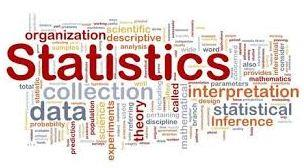Let us remember that Mean, Median and Mode are measures of central tendency i.e., numerical representatives of the given data. Let us also note the following points.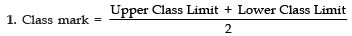2. Mean of grouped data:
(i) Using direct method: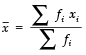(ii) Using the assumed mean method: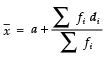(iii) Using the step deviation method: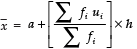3. The mode for grouped data is found by using the formula: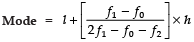where, l = lower limit of the modal class.
h = size of the class interval.
f1 = frequency of the modal class.
f0 = frequency of the class preceding the modal class.
f2 = frequency of the class succeeding the modal class.4. Cumulative frequency of a class is the frequency obtained by adding the frequencies of all the classes preceding the given class.

5. Median of the given grouped data is obtained using the formula: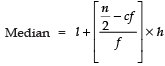where, l = lower limit of median class.
n = number of observations.
cf = cumulative frequency of class preceding the median class
f = frequency of median class
h = class size

Offer running on EduRev: Apply code STAYHOME200 to get INR 200 off on our premium plan EduRev Infinity!

132 docs

,

,

,

,

,

,

,

,

,

,

,

,

,

,

,

,

,

,

,

,

,

;